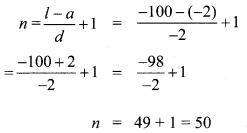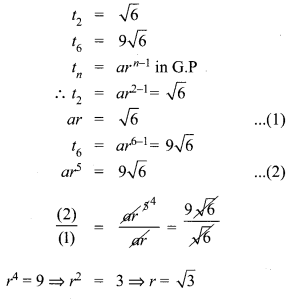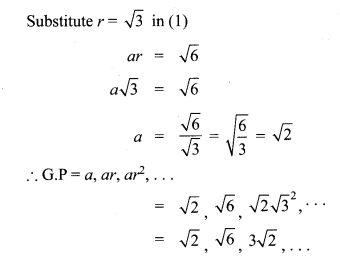# Tamilnadu Board Class 10 Maths Solutions Chapter 2 Numbers and Sequences Unit Exercise 2

## Tamilnadu State Board Class 10 Maths Solutions Chapter 2 Numbers and Sequences Unit Exercise 2

Question 1.
Prove that n2 – n divisible by 2 for every positive integer n.
To prove n2 – n divisible by 2 for every positive integer n.
We know that any positive integer is of the form 2q or 2q + 1, for some integer q.
So, following cases arise:
Case I. When n = 2q.
In this case, we have
n2 – n = (2q)2 – 2q = 4q2 – 2q = 2q(2q – 1)
⇒ n2 – n = 2r where r = q(2q – 1)
⇒ n2 – n is divisible by 2.

Case II. When n = 2q + 1.
In this case, we have
n2 – n = (2q + 1)2 – (2q + 1)
= (2q + 1)(2g + 1 – 1) = (2q + 1)2q
⇒ n2 – n = 2r where r = q (2q + 1)
⇒ n2 – n is divisible by 2.
Hence n2 – n is divisible by 2 for every positive integer n.

Question 2.
A milk man has 175 litres of cow’s milk and 105 litres of buffalow’s milk. He wishes to sell the milk by filling the two types of milk in cans of equal capacity. Calculate the following (i) Capacity of a can (ii) Number of cans of cow’s milk (iii) Number of cans of buffalow’s milk.
175 litres of cow’s milk.
105 litres of goat’s milk.
H.C.F of 175 & 105 by using Euclid’s division algorithm.
175 = 105 × 1 + 70, the remainder 70 ≠ 0
Again using division algorithm,
105 = 70 × 1 + 35, the remainder 35 ≠ 0
Again using division algorithm.
70 = 35 × 2 + 0, the remainder is 0.
∴ 35 is the H.C.F of 175 & 105.
(i) ∴ The milk man’s milk can’s capacity is 35 litres.
(ii) No. of cow’s milk obtained = $$\frac { 175 }{ 35 }$$ = 5 cans
(iii) No. of buffalow’s milk obtained = $$\frac { 105 }{ 35 }$$ = 3 cans

Question 3.
When the positive integers a, b and c are divided by 13 the respective remainders are 9, 7 and 10. Find the remainder when a + 2b + 3c is divided by 13.
Let the positive integers be a, b, and c.
a = 13q + 9
b = 13q + 7
c = 13q + 10
a + 2b + 3c = 13 q + 9 + 2(13q + 7) + 3(13q + 10)
= 13q + 9 + 269 + 14 + 39q + 30
= 78q + 53 = (13 × 6)q + 53
The remainder is 53.
But 53 = 13 × 4 + 1
∴ The remainder is 1

Question 4.
Show that 107 is of the form 4q +3 for any integer q.
Solution:
107 = 4 × 26 + 3. This is of the form a = bq + r. Hence it is proved.

Question 5.
If (m + 1)th term of an A.P. is twice the (n + 1)th term, then prove that (3m + 1)th term is twice the (m + n + 1)th term.
Solution:
tn = a + (n – 1)d
tm+1 = a + (m + 1 – 1)d
= a + md
tn+1 = a + (n + 1 – 1)d
= a + nd
2(tn+1) = 2(a + nd)
tm+1 = 2tn+1 ….(1)
⇒ a + md = 2(a + nd)
2a + 2nd – a – md = 0
a + (2n – m)d = 0
t(3m+1) = a + (3m + 1 – 1)d
= a + 3md
t(m+n+1) = a + (m + n + 1 – 1)d
= a + (m + n)d
2(t(m+n+1)) = 2(a + (m + n)d)
= 2a + 2md + 2nd
t(3m+1) = 2t(m+n+1) …(2)
a + 3md = 2a + 2md + 2nd
2a + 2md + 2nd – a -3md = 0
a – md + 2nd = 0
a + (2n – m)d = 0
∴ It is proved that t(3m+1) = 2t(m+n+1)

Question 6.
Find the 12th term from the last term of the A.P -2, -4, -6,… -100.
Solution:12th term from the last
= 39th term from the beginning
∴ t39 = a + 38d
= -2 + 38(-2)
= – 2 – 76
= – 78

Question 7.
Two A.P’s have the same common difference. The first term of one A.P is 2 and that of the other is 7. Show that the difference between their 10th terms is the same as the difference between their 21st terms, which is the same as the difference between any two corresponding terms.
Solution:
Let the two A.Ps be
AP1 = a1, a1 + d, a1 + 2d,…
AP2 = a2, a2 + d, a2 + 2d,…
In AP1 we have a1 = 2
In AP2 we have a2 = 7
t10 in AP1 = a1 + 9d = 2 + 9d ….(1)
t10 in AP2 = a2 + 9d = 7 + 9d …..(2)
The difference between their 10th terms
= (1) – (2) = 2 + 9d – 7 – 9d
= -5 ….(I)
t21 m AP1 = a1 + 20d = 2 + 20d …(3)
t21 in AP2 = a2 + 20d = 7 + 20d …(4)
The difference between their 21 st terms is
(3) – (4)
= 2 + 20d – 7 – 20d
= -5 ….(II)
I = II
Hence it is Proved.

Question 8.
A man saved ₹16500 in ten years. In each year after the first he saved ₹100 more than he did in the preceding year. How much did he save in the first year?
Solution:
S10 = ₹16500
a, a + d, a + 2d…
d = 100
n = 10
Sn = $$\frac { n }{ 2 }$$(2a+(n-1)d)
S10 = 16500
S10 = $$\frac { 10 }{ 2 }$$(2×a+9×100)
16500 = 5(2a+900)
16500 = 10a + 4500
10a = 16500 – 4500
10a = 12000
a = $$\frac { 12000 }{ 10 }$$ = ₹1200
∴ He saved ₹1200 in the first year

Question 9.
Find the G.P. in which the 2nd term is $$\sqrt { 6 }$$ and the 6th term is 9$$\sqrt { 6 }$$.
Solution:Question 10.
The value of a motor cycle depreciates at the rate of 15% per year. What will be the value of the motor cycle 3 year hence, which is now purchased for ₹45,000?
Solution:
a = ₹45000
Depreciation = 15% for ₹45000
= 45000 × $$\frac { 15 }{ 100 }$$
d = ₹6750 since it is depreciation
d = -6750
At the end of 1st year its value = ₹45000 – ₹6750
= ₹38250,
Again depreciation = 38250 × $$\frac { 15 }{ 100 }$$ = 5737.50
At the end of 2nd year its value
= ₹38250 – ₹5737.50 = 32512.50
Again depreciation = 32512.50 × $$\frac { 15 }{ 100 }$$ = 4876.88
At the end of the 3rd year its value
= 32512.50 – 4876.88 = 27635.63
∴ The value of the automobile at the 3rd year
= ₹ 27636